# Finish up Lecture 3 slides

## Sampling distributions

Options for finding the sampling distribution:

• Derive it mathematically
• Can’t derive the distribution?
• Derive properties of the distribution
• Simulate
• Approximate

# Deriving the sampling distribution

## Normal population: set up

Population distribution: $$Y \sim N(\mu, \sigma^2)$$

Sample: $$Y_1, \ldots, Y_n$$ i.i.d from population

Sample statistic: Sample mean = $$\overline{Y} = \frac{1}{n}\sum_{i=1}^n Y_i$$

What is the sampling distribution of the sample mean?

## Normal population: derivation

$$Y_1 + Y_2 \sim$$

$$Y_1 + Y_2 + Y_3 \sim$$

$$Y_1 + Y_2 + \ldots + Y_n \sim$$

$$\overline{Y} = \frac{Y_1 + Y_2 + \ldots + Y_n}{n} \sim$$

## Bernoulli population

Population distribution: $$Y \sim \textit{Bernoulli}(p)$$

E.g US voters where $Y = \begin{cases} 1, & \text{Supports single payer health care} \\ 0, & \text{Does not support single payer health care} \end{cases}$

Sample: $$Y_1, \ldots, Y_n$$, i.i.d from population

Sample Statistic: Sample mean = $$\overline{Y} = \frac{1}{n}\sum_{i=1}^n Y_i =$$ gives the sample proportion

What is the sampling distribution of the sample proportion? $$\sum_{i=1}^n Y_i \sim \text{Binomial} (n, p)$$

## Bernoulli population

E.g. $$p = 0.56$$, $$n = 10$$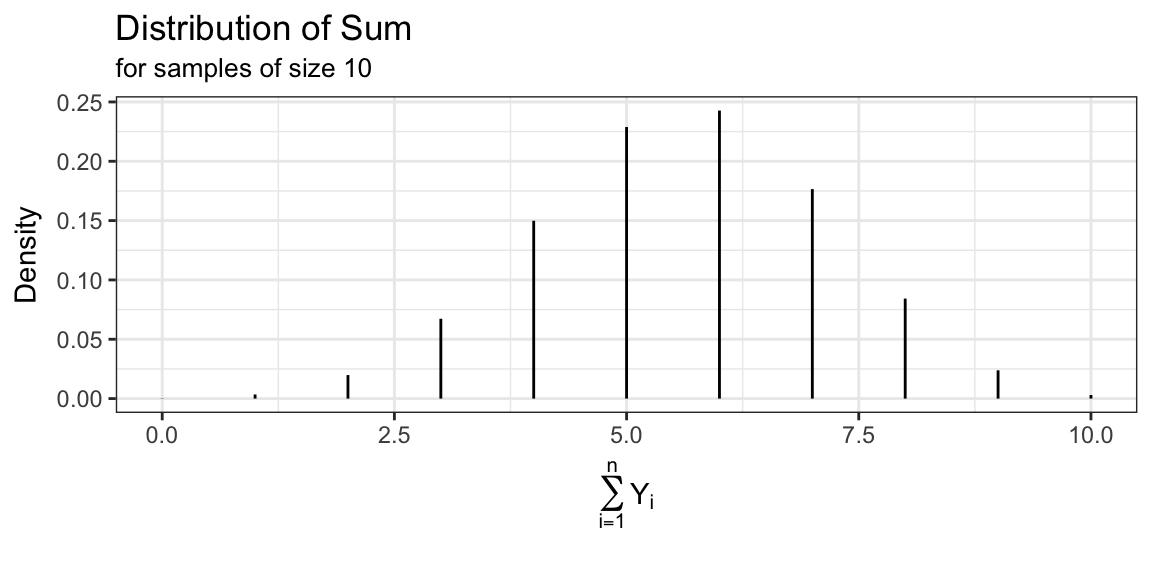## Bernoulli population

E.g. $$p = 0.56$$, $$n = 10$$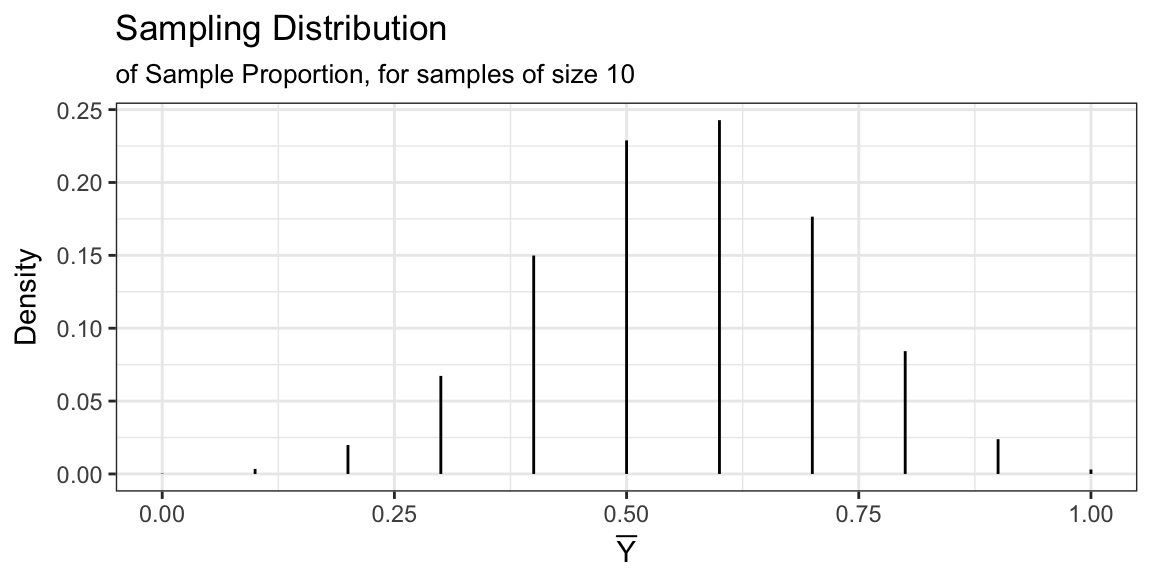## Can’t derive in these situations

• Population: $$Y \sim Uniform(a, b)$$
• Sample: size $$n$$ i.i.d
• Statistic: sample mean or sample variance
• No closed form solution
• Population $$Y \sim$$ unknown
• Sample: size $$n$$ i.i.d
• Statistic: anything
• Can’t derive because we don’t know population distribution

## What to do?

1. Derive parameters of sampling distribution
2. Simulate the sampling distribution
3. Approximate the sampling distribution

# Some more probability review

## Cumulative Density Function

The cumulative density function of a random variable $$X$$ is $F(x) = P(X \le x)$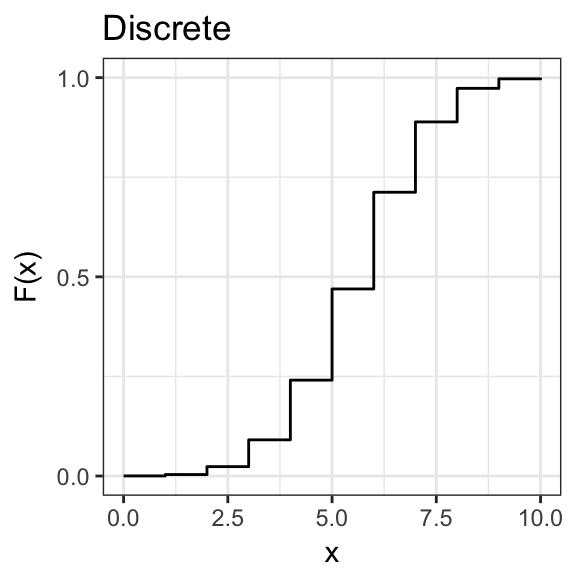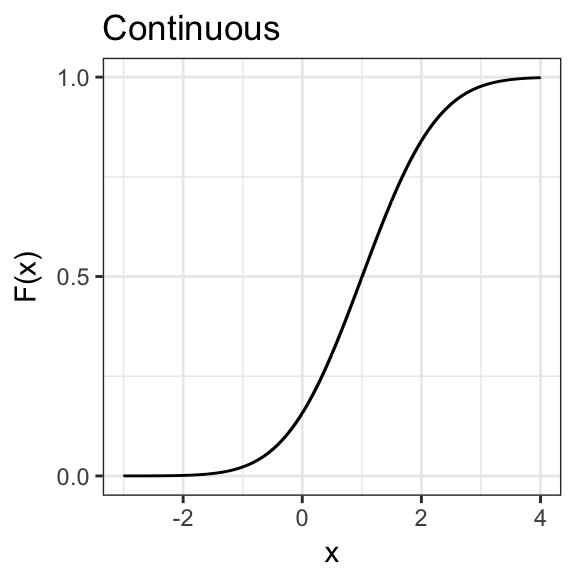## Probabilty Density/Mass Function

For continuous distributions we can define the probability density function:

$f(x) = \frac{d}{dx}F(x) \approx \frac{P\left(X \in (x - \Delta,\, x + \Delta) \right)}{2\Delta}$ For discrete distributions we have probability mass function:

$p(x) = P(X = x)$

## Probabilty Density/Mass Function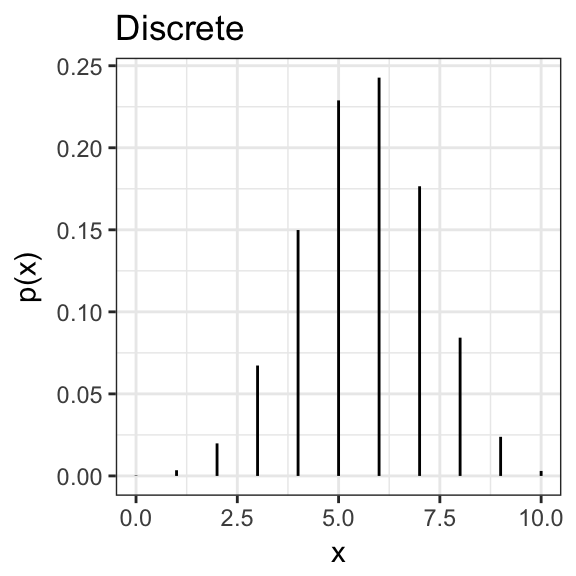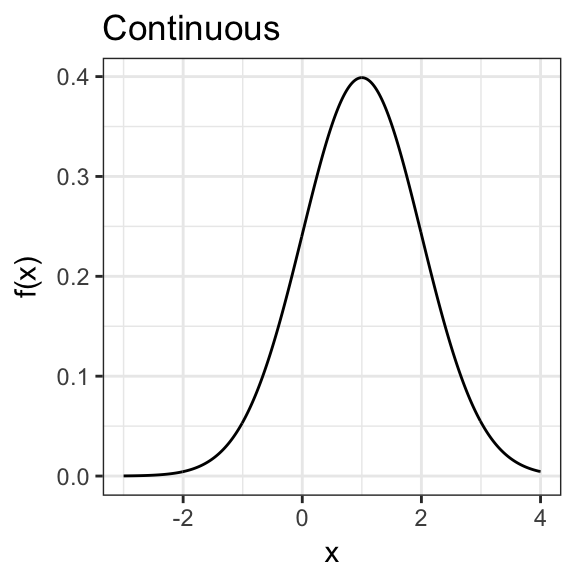## Expectation (Mean)

The expectation (or mean) of a random variable, $$X$$, is

\begin{aligned} E(X) &= \int\limits_{-\infty}^{\infty}xf(x) dx \quad &\text{for continuous distributions}\\ E(X) &= \sum\limits_{x: p(x) > 0} x p(x) \quad &\text{for discrete distributions} \end{aligned}

## Expectation Properties

For any random variables $$X$$ and $$Y$$ (don’t need independence)

$$E(X + Y) = E(X) + E(Y)$$

$$E(a_1X_1 + \ldots + a_nX_n) = a_1E(X_1) + \ldots + a_nE(X_n)$$

Known as the linearity property.

## Variance and Covariance

The variance of r.v. $$X$$ is $Var(X) = E\bigl[ \left(X - E(X)\right)^2 \bigr] = E[X^2] - \left(E[X]\right)^2$

The covariance between r.v.’s $$X$$ and $$Y$$ is $Cov(X, Y) = E\left[\bigl(X- E(X) \bigr)\bigl(Y - E(Y) \bigr)\right]$ If $$X$$ and $$Y$$ are independent $$Cov(X, Y) = 0$$ (converse isn’t true)

$$Cov(X, X) = Var(X)$$

## Variance Properties

For any random variables $$X$$ and $$Y$$ (don’t need independence)

$$Var(X + Y) = Var(X) + Var(Y) + 2Cov(X, Y)$$

$$Var(X - Y) = Var(X) + Var(Y) - 2Cov(X, Y)$$

For random variables $$X_1, \ldots, X_n$$ \begin{aligned} \textit{Var}& \left(a_1 X_1 + \ldots + a_n X_n \right)= a_1^2 \textit{Var}(X_1) + \ldots + a_n^2 \textit{Var}(X_n) \, +\\ & a_1a_2 \textit{Cov}(X_1, X_2) + a_1a_3\textit{Cov}(X_1, X_3) + \ldots + a_1a_n\textit{Cov}(X_1, X_n) \, + \\ & \vdots \\ & a_na_1\textit{Cov}(X_n, X_1) + a_na_2\textit{Cov}(X_n, X_2) + \ldots + a_na_{n-1}\textit{Cov}(X_n, X_{n-1}) \end{aligned}

## Next time…

Use these properties to derive mean and variance for sampling distributions.